#2018 iT 邦幫忙鐵人賽DAY 21
0
Software Development

# 可失敗初始化器 (Failable Initializers)

``````let number1 = 87.0
if let valueChange = Int(exactly: number1){
print("數字 1 : \(number1) 可以保持原有的值，轉換成 Int 。")
}

let number2 = 87.2
let valueNil = Int(exactly: number2)
if valueNil == nil {
print("數字 2 : \(number2) 無法保持原值轉換類型。")
}
``````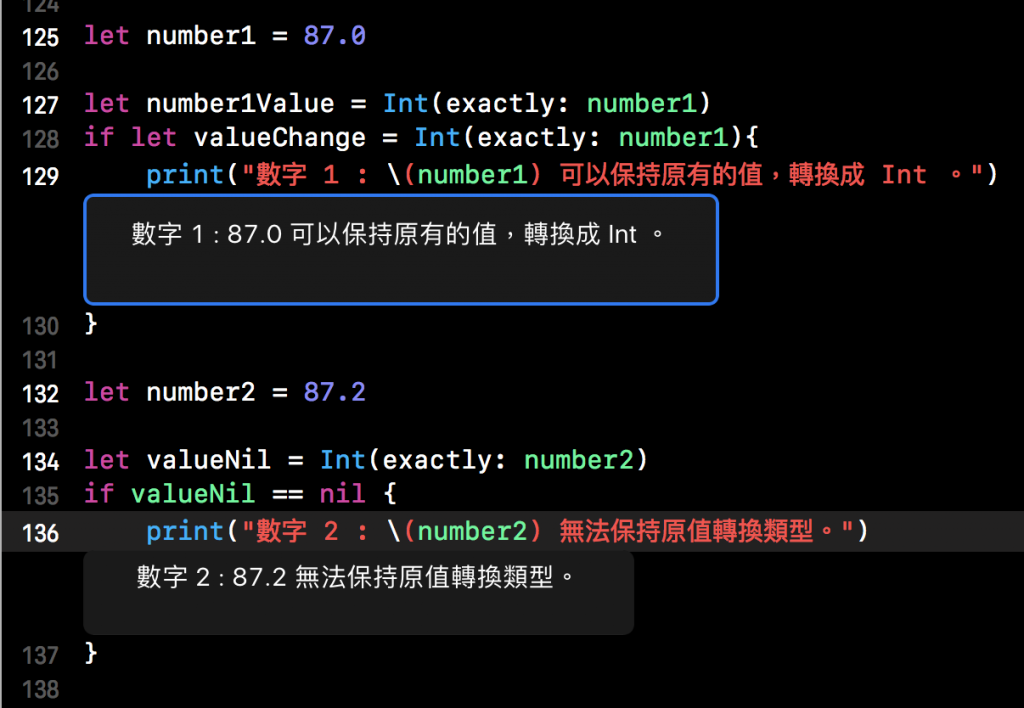``````struct Student {
let name:String
init?(name:String) {
if name.isEmpty {return nil}
self.name = name
}
}
``````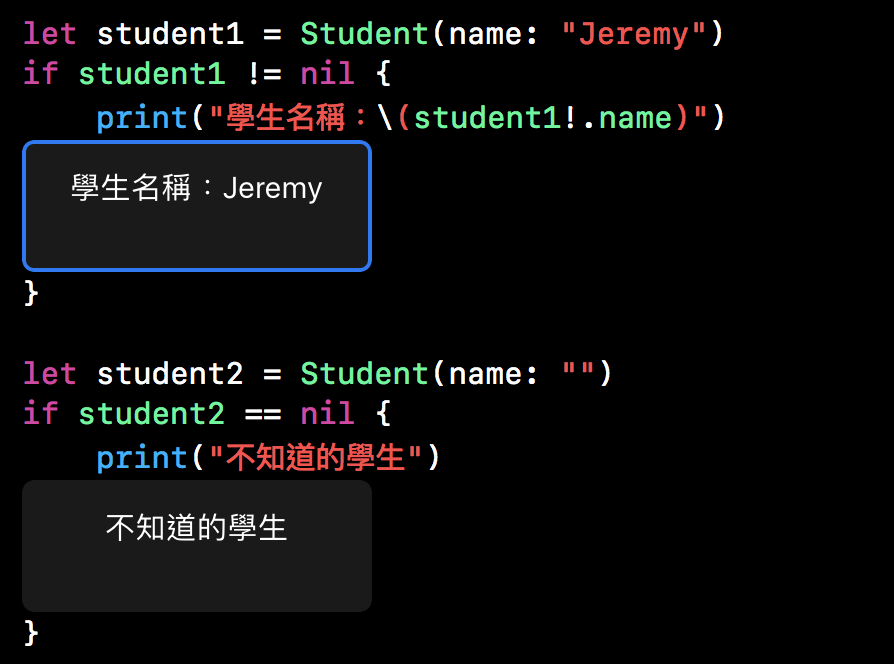# 枚舉的可失敗初始化器

``````enum Restaurant {
case food, soup, drink
init?(item: String){
switch item {
case "食物":
self = .food
case "湯品":
self = .soup
case "飲料":
self = .drink
default:
return nil
}
}
}
``````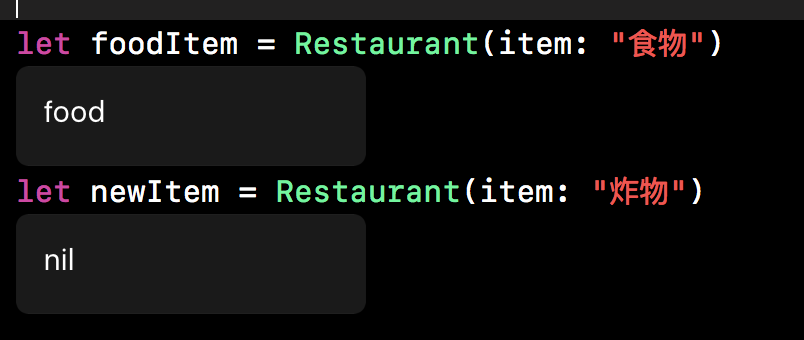# 帶有原始值枚舉的可失敗初始化器

``````enum Restaurant: String {
case food = "食物", soup = "湯品", drink = "飲料"
}
``````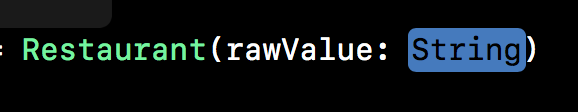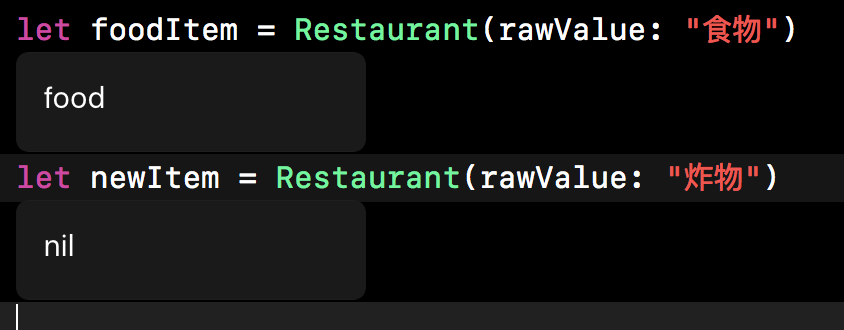# 初始化失敗的傳遞

Class，Struct 或 enum 的可失敗初始化器可以橫向委託到同一個 Class 中、Struct 或 enum 裡的另一個可失敗初始化器。同樣的，子類的可失敗初始化器可以向上委託到父類的可失敗初始化器。無論哪種情況，如果你委託到另一個初始化器導致了初始化失敗，那麼整個初始化過程也會跟著失敗，並且之後任何初始化都不會執行。

``````class Classes {
let className:String
init?(className:String) {
if className.isEmpty {return nil}
self.className = className
}
}

class Student:Classes {
let student:Int
init?(className:String, student:Int) {
if student < 1 { return nil}
self.student = student
super.init(className: className)
}
}
``````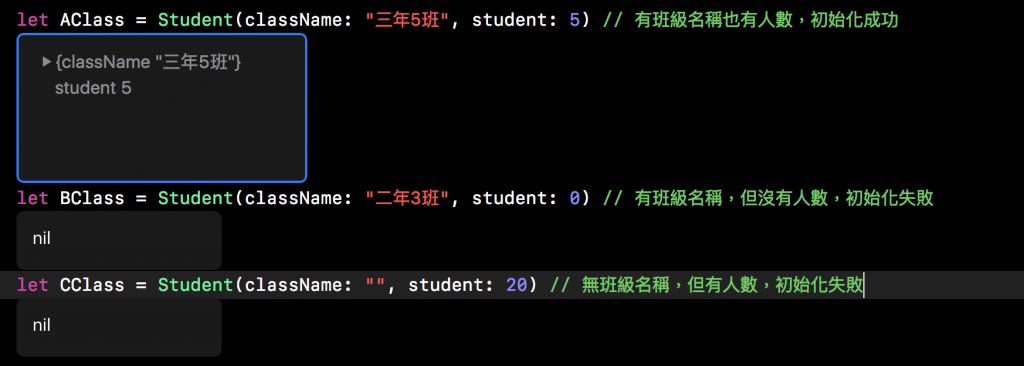# 重寫可失敗初始化器

``````class File {
var name:String?
init() {}
init?(name:String) {
self.name = name
if name.isEmpty {return nil}
}
}
``````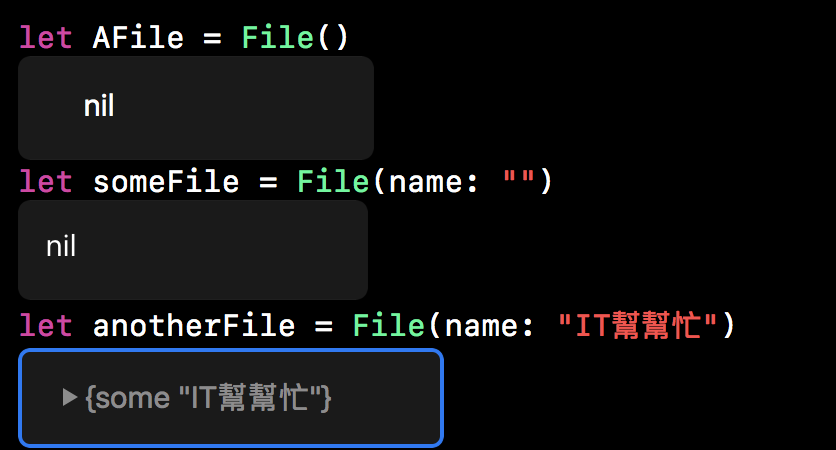``````class AutoName:File {
override init() {
super.init()
self.name = "未命名文件"
}
override init?(name: String) {
super.init()
if name.isEmpty {
self.name = "未命名文件"
} else {
self.name = name
}
}
}
``````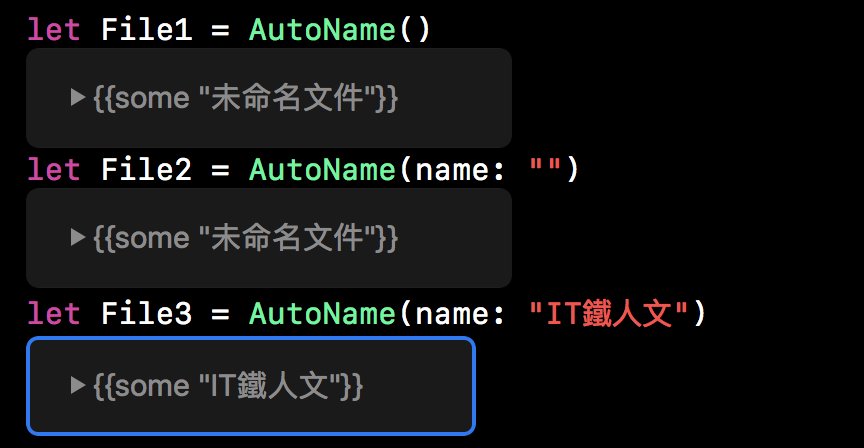``````class Unknow:File {
override init() {
super.init(name: "未命名文件")!
}
}
``````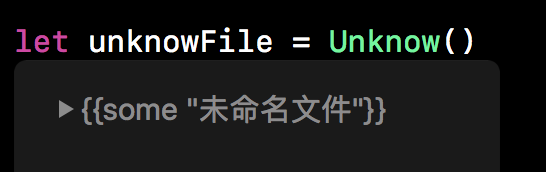# 必要初始化器

``````class SomeClass {
required init() {
}
}
``````

``````class SomeSubclass: SomeClass {
required init() {
// subclass implementation of the required initializer goes here
}
}
``````

Swift 菜鳥的30天30